Palma, S., and Gonçalves, R. (2022). "Tomographic images of tree trunks generated using ultrasound and post-processed images: Influence of the number of measurement points," BioResources 17(4), 6638-6655.

#### Abstract

Tomography is a technique increasingly used in tree inspections. This technique can be performed in two stages: field testing and postimage processing. To feed the tomographic image construction software, it is necessary to adopt a measurement grid composed of points positioned on the perimeter of the stem. The images are generated through spatial interpolation algorithms. From a theoretical point of view, more measurement points taken in the perimeter of the stem result in better interpolation results. However, an increase in the number of mesh points causes a substantial increase in field work and image processing time. The general objective of this study was to verify the influence of the number of measurement points of the diffraction mesh on the ultrasound tomography results. For this purpose, ten simulated discs were used, all 500 mm in diameter and with different defects in terms of size, position, and geometry. In each of the discs, diffraction grids were simulated with 6, 8, 10, 12, and 14 measurement points in the contour. The results showed that a favorable combination of accuracy and a minimization of effort can be achieved with diffraction mesh with number of measurement points calculated as five times the perimeter of the trunk in meters.

Tomographic Images of Tree Trunks Generated Using Ultrasound and Post-processed Images: Influence of the Number of Measurement Points

Stella S. A. Palma,a and Raquel Gonçalves a,*

Tomography is a technique increasingly used in tree inspections. This technique can be performed in two stages: field testing and postimage processing. To feed the tomographic image construction software, it is necessary to adopt a measurement grid composed of points positioned on the perimeter of the stem. The images are generated through spatial interpolation algorithms. From a theoretical point of view, more measurement points taken in the perimeter of the stem result in better interpolation results. However, an increase in the number of mesh points causes a substantial increase in field work and image processing time. The general objective of this study was to verify the influence of the number of measurement points of the diffraction mesh on the ultrasound tomography results. For this purpose, ten simulated discs were used, all 500 mm in diameter and with different defects in terms of size, position, and geometry. In each of the discs, diffraction grids were simulated with 6, 8, 10, 12, and 14 measurement points in the contour. The results showed that a favorable combination of accuracy and a minimization of effort can be achieved with diffraction mesh with number of measurement points calculated as five times the perimeter of the trunk in meters.

DOI: 10.15376/biores.17.4.6638-6655

Keywords: Tree inspection; Quality of tomography in trees; Measuring mesh in trunks

Contact information: School of Agricultural Engineering – University of Campinas, Campinas, São Paulo, Brazil; *Corresponding author: raquelg@unicamp.br

INTRODUCTION

The importance of trees in the urban environment is widely recognized. Despite their numerous benefits, trees can also generate problems due to phytosanitary conditions (Wolf et al. 2020). Urban conditions (lack of space, waterproofing and compaction of the terrain, competition with urban equipment, etc.) are, in general, aggressive to living beings, making them more susceptible to falls (Czaja et al. 2020). This condition is further aggravated in some countries due to the lack of planning in the choice of species, lack of care in the planting bed, and in silvicultural treatments, such as inadequate pruning that favor the action of rotting fungi due to the occurrence of necrosis in the trunks (Suchocka et al. 2021). To avoid falls, especially from the action of winds, inspections are important. Among the methodologies used in tree inspections, acoustic tomography is very promising (Gilbert et al. 2016; Palma et al. 2018; Strobel et al. 2018; Espinosa et al. 2020, Dudkiewicz and Durlak 2021; Linhares et al. 2021).

To prepare the tomographic images, wave propagation time measurements are performed using a mesh. In this mesh, the emitter sensor is located at each node while the receiving sensor travels through the other nodes. This mesh produces theoretical wave propagation routes where the velocity is calculated using the relationship between the theoretical distance traveled by the wave and the propagation time. For the preparation of the tomographic image, wave propagation speed ranges are associated with colors. A thinner mesh produces a greater scope for the inspection inside the inspected element, as there will be a greater number of measurement routes (Divos and Divos 2005; Zhang and Khoshelham 2020; Espinosa et al. 2020; Dudkiewicz and Durlak 2021), allowing the visualization of deteriorations in early stages (Gilbert et al. 2016). However, increasing the number of theoretical measurement routes implies increasing the measurement points in the stem contour, which consequently increases field work (Espinosa et al. 2020). As an example, a diffraction mesh such as the one used in this study, with six measurement points in the stem contour and measurements made in only one direction (e.g., 1 for 2 or 2 for 1), results in 15 routes to be measured in the tree (Fig. 1). The number of routes increases to 28 when there are eight measurement points in the contour (Fig. 1), 45 when there are ten measurement points, 66 with 12 measurement points, and 91 with 14 measurement points.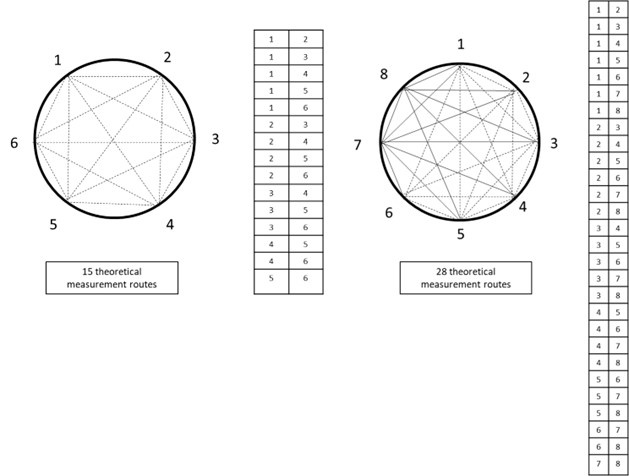Fig. 1. Example of theoretical wave propagation routes in diffraction grids with six and eight measurement points in the contour of a stem

Even with a very fine mesh (with many measurement points in the contour of the stem), there will be internal zones of the stem through which no theoretical routes pass, and therefore, there is no calculated wave propagation speed (Feng et al. 2014). For this reason, for the preparation of a tomographic image, it is necessary to associate the pixels with velocity values obtained by interpolation, for which different algorithms are adopted (Feng et al. 2014; Du et al. 2015, 2018, Strobel et al. 2018). The increase in the number of measurement points causes the interpolation to be performed with pixels that are closer to the theoretical routes and have calculated velocity values. Therefore, an improvement in the quality of the inference of velocities obtained through the interpolation is expected (Gilbert et al. 2016). However, the increase in image quality is not proportional to the increase in the number of measurement points in the mesh (Divos and Divos 2005) and causes an increase in field work and image processing time.

The quality of the tomographic image can also be improved through filters, which can be applied to reduce interference from various causes (Pratt 1978). Filters applied to the signal are common in ultrasound equipment, even in the simplest ones, such as the one used in this study. Moreover, in more sophisticated ultrasonic tomography technologies, filters are applied to the signal after its passage in the material, which reduces the specific noise that occurs in the propagation during inspection, as well as separates the desired signal ranges (Espinosa et al. 2020). A simple way to minimize interference is the use of filters applied directly to the images to smooth effects from interpolation systems, including simplified techniques such as the median filter (Sun and Neuvo 1994).

Many studies that seek to evaluate the interference of certain factors in acoustic tomography images are based on the visual comparison of images and are therefore subjective. There are tools that can make these evaluations more objective, including the confusion matrix, with which the comparison is made by metrics (Strobel et al. 2018).

Based on the analysis of the velocities in the theoretical measurement routes and in the metrics of the confusion matrix, this study proposes a minimum number of measurement points in the diffraction mesh that does not affect the quality of the ultrasound tomography images.

EXPERIMENTAL

Simulations

The analysis was performed using ten simulated discs, all 500 mm in diameter and with ten different defects in terms of size, position, and geometry (Fig. 2). Simulation, although it is a simplification of reality, is an important tool to isolate a given analysis from interferences that are not desired or cannot be measured. In the case of this research, the simulation made it possible to avoid interferences from wood heterogeneity in the process of analyzing the mesh measurement routes. In each disc, diffraction grids were simulated with 6, 8, 10, 12, and 14 measurement points in the contour (Fig. 3). The use of diffraction grids with an even number of measurement points allows the occurrence of three types of routes: radial, internal tangential, and edge tangential. The image of the discs with defects (Fig. 2) and the overlap of the diffraction grids with different numbers of points (Fig. 3) were made with the image processing software ImageJ (version 1.51), which has an open architecture. Using ImageJ, the 400 x 400-pixel units of the images were scaled to centimeters. Considering that the disc was simulated with a circular shape, one of the radial routes (diagonal) was selected with the “straight line” tool to obtain a referential dimension in pixels. Then, with the “set scale” tool, the conversion was performed, assigning 50 cm to the value obtained in pixels. Then, the stretch of interest (route that passed through the defect) was marked, and its length was obtained using the “straight line” (ImageJ) and “measure” (ImageJ) commands, respectively. This procedure was performed for each theoretical measurement route that passed through the defect, considering all the conditions of the number of measurement points adopted in the study (6, 8, 10, 12, and 14). The theoretical path lengths were obtained within the zone with the simulated defect, which were called (L c), adopting the simulated defect as a cavity.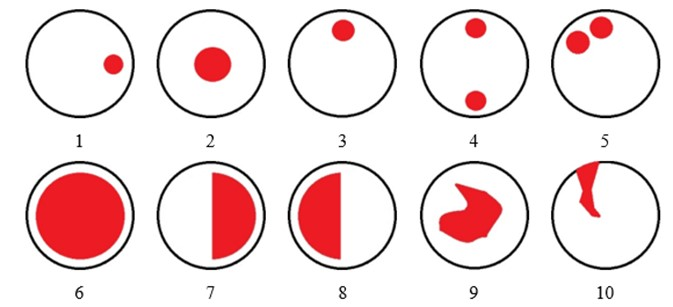Fig. 2. Scheme of the ten simulated defects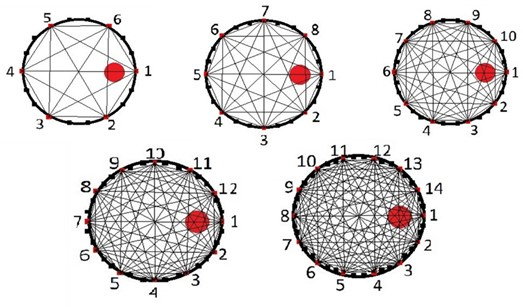Fig. 3. Example of a diffraction mesh with 6, 8, 10, 12 and 14 points in the contour with representation of the respective theoretical measurement routes considering a lateral defect

Methods

Generation of images

The image was generated using the ImageWood (version 3.1), software developed by the Nondestructive Testing Research Group (School of Agricultural Engineering – University of Campinas, Brazil). To use this image generator software (ImageWood), it is necessary to feed the system with two spreadsheets, the contour spreadsheet and the time spreadsheet. The contour spreadsheet contains the coordinates of the contour, and the time spreadsheet contains the initial and final coordinates of the measurement route associated with the respective time obtained by the wave propagation test.

The contour worksheet contains the position, in a 2D plane, of the coordinates (x, y) of the perimeter points of the disc (contour) and, among them, the coordinates of each point, where in a real inspection, the transducer would be a coupled emitter (xi, yi) and receiver (xf, yf). The time sheet is obtained from a combination of the coordinates of the measurement points (xi, yi and xf, yf) by generating the theoretical wave propagation route to which the propagation time is assigned.

Considering that all the simulated discs had a known diameter (500 mm) and had perfectly circular circumferences, the perimeter coordinates for feeding the contour spreadsheet were calculated using a macro (regular contour generator – GCR) developed for ImageJ per student in the group of research in the Scientific Initiation program of the University. This macro allows one to obtain the coordinates of the contour, among which are the measurement points. The software is free available in the University repository (https://doi.org/10.25824/redu/SEJFAC). The macro also allows saving the points automatically in a text file. Thus, to obtain the time sheet, the initial and final coordinates of the points corresponding to the ends of each measurement route were adopted.

However, to complete the time sheet, it was necessary to insert the wave propagation times into the theoretical routes. As the discs were simulated, there were no real velocity measurements. Thus, it was necessary to propose a methodology to simulate the wave behavior and determine the final velocities in the theoretical propagation routes.

During a parallel survey conducted with inspection of real discs containing different types and dimensions of cavities (Palma 2022 – Article 2), a model of variation of the percentage of maximum velocity was obtained from an index (Ic) corresponding to the path length within the zone with cavity (Lc), as shown in Eq. 1.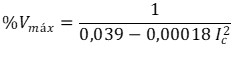(1)

In Eq. 1, Ic = 0 when Lc corresponds to 100% of the interval; Ic = 1 when Lc is between 91% and 100% of the interval; Ic = 2 when Lc is between 80% and 90% of the interval; Ic = 3 when Lc is between 70% and 80% of the interval; Ic = 4 when Lc is between 60% and 70% of the interval; Ic = 5 when Lc is between 50% and 60% of the interval; Ic = 6 when Lc is between 40% and 50% of the interval; Ic = 7 when Lc is between 30% and 40% of the interval; Ic = 8 when Lc is between 20% and 30% of the interval; Ic = 9 when Lc is between 10% and 20% of the interval; and Ic = 10 when Lc <10% of the interval. The model was statistically significant with a 95% confidence level and coefficient of determination R2 = 79%.

As the defects are simulated and the propagation medium was considered perfect in this study, the speed in all routes that did not pass through the defect was considered constant with a value of 2000 m/s, compatible with the order of magnitude of the means of velocities perpendicular to the fibers in intact wood. Thus, the speeds in the routes that passed by default were obtained by applying the percentage of maximum speed (%Vmax) obtained with Eq. 1 to the value of the maximum speed (2000 m/s).

With the contour and time sheets, the image generator software (ImageWood) automatically calculated the theoretical lengths and velocities in the theoretical wave propagation routes. However, when simulating this propagation, the time was calculated as a function of the simulated speed in the routes without defects (2000 m/s) and with defects (calculated with Eq. 1). Thus, with the initial (xi, yi) and final (xj, yj) coordinates of the theoretical routes, the theoretical distances (L) traveled by the wave were calculated using (Eq. 2) in Excel.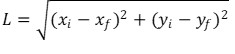(2)

Finally, with the simulated velocities and theoretical path lengths, the simulated propagation times were calculated and entered in the time sheet to feed the ImageWood software.

For the formation of the tomographic image, it was also necessary to adopt a cutoff value of the percentage of the maximum velocity that would allow a better representation of the presence of the defect in an image. It is known that in the presence of cavities, the wave propagation velocity decreases (Lin et al. 2008; Palma 2017, Du et al. 2018). In previous studies of the group (Palma 2017; Reis 2022), the most adequate cutting speed for cavity zones was 40% of the maximum speed; this value was adopted for this study.

The software used for image generation, ImageWood, allows the use of two spatial interpolation algorithms: the inverse square distance (ISD) and the methods of ellipses (ME). Although previous research by the group showed good results for ME (Palma et al. 2018; Strobel et al. 2018; Reis 2022), the ME method always changes the calculated velocities with the measured real times, since the measurement route, theoretically adopted as a straight line, is transformed into an ellipse. The transformation increases the path and, as the measured real time remains, the velocity also increases proportionally (Du et al. 2015). Considering that in this study it is necessary that the simulated velocity for the intact zone be kept fixed at 2000 m/s, the DQI method was adopted.

Image filtering

Images generated by the ISD algorithm show interference in the form of cords that radiate from the zone in which the velocities differ (Palma 2017). To reduce these interferences, the use of filters was tested. Before deciding on the filter to be used in the continuation of the research, several filters were tested using imageJ. It is important to highlight that filters are need to smooth the edges. This is because, in this interpolation algorithm (ISD), the images assume the shape of the mesh (polygons). The preliminary tested filters and the summary of the results that implied in their discards from the rest of the analysis were “Convolver”, “Variance” and “Unsharp mask” because they marked or reinforced the contour instead of softening it; “Gaussian blur” and “Mean” because they blurred the image; and “Minimum” and “Maximum” because they decreased and increased the image, respectively. The “Median” was the filter that presented the better result and, due to this result, it was adopted in the rest of the research. The median filter mitigates impulsive noise, replacing the intensity of each pixel by the median of the neighboring intensities. For this, the images of each simulated disc were generated for each condition of number of measurement points (6, 8, 10, 12, 14) without and with the application of a median filter, totaling 100 images (10 simulated discs x 5 conditions of number of measuring points x 2 conditions (with and without filter)). In an iterative manner, each image was visually evaluated, comparing it with the simulated conditions (Fig. 2), until for each condition of number of measurement points, the ideal number of neighboring pixels to filter the image was obtained.

Image evaluation

After the adoption of the filters, the final ultrasound tomography images were generated using the IQD interpolator for the ten discs with simulated defects and with diffraction grids with 6, 8, 10, 12 and 14 measurement points in the contour. The confusion matrix was used to assess how much the increase in the number of measurement points interferes with the quality of the tomographic image. This evaluation consists of comparing two images using the metrics of accuracy, precision, recall, and F1-score. For this purpose, a binary confusion matrix method was used, with the Python script proposed by Strobel et al. (2018). For the comparison using the confusion matrix, it is necessary to have three types of disc images named by the method as Roi (region of interest), model, and internal points. The three images must be binary and of the same size in pixels, having been treated in the ImageJ software. The Roi or region of interest was obtained from the binarization of the image, generated by ImageWood with only two colors (up to 40% of the maximum speed was associated with yellow and above 40% of the maximum speed was associated with brown) and filtered. From this image, adjusted to 400 x 400 pixels, Roi was obtained, highlighting only the region of interest, which in this case was the region in yellow obtained by the tomographic image in the inference of the defect (Example in Fig. 4).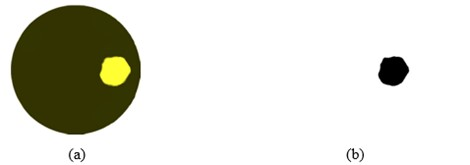Fig. 4. Example of Roi (b) generated from tomographic image (a) of the defect

The model is the reference for the evaluation of tomographic images. To obtain this reference, the resolution of the images of the ten simulated discs (Fig. 2) were adjusted to the same dimension (400 x 400 pixels) used in the tomographic images, and through binarization, the defect was highlighted (Fig. 5).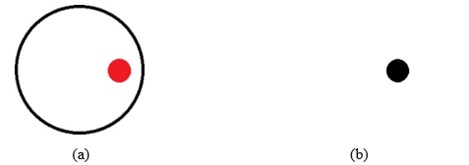Fig. 5. Example of model (b) obtained from the image of the simulated defect (a).

The image of internal points corresponds to the image formed by the delimitation of the field where the comparison of the model with Roi should occur. This image is obtained from the inverted binarization of the tomographic image, highlighting the area outside the contour (Example in Fig. 6).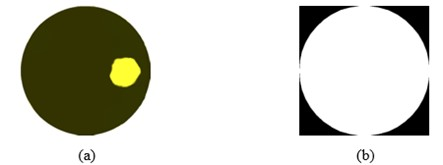Fig. 6. Example of internal points (b) generated from the tomographic image of the simulated defect (a)

The evaluation of image quality was performed by comparing the pixels within the internal points using the combinations proposed by (Fawcett 2006). The pixels within the zone of interest (in the case of this study, the zone with defects) were considered positive and pixels outside the zone of interest were considered negative.

• True positive (PV): pixels which were expected to be positive and the result was positive;
• False negative (FN): pixels which were expected to be positive and the result was negative;
• True negative (NV): pixels which were expected to be negative and the result was negative;
• False-positive (FP): pixels which were expected to have a negative result and the result was positive.

With the results of the combinations, different metrics can be calculated (Luque et al. 2019), from which the accuracy (Eq. 3), precision (Eq. 4), recall (Eq. 5) and F1-score were used. (Eq. 6).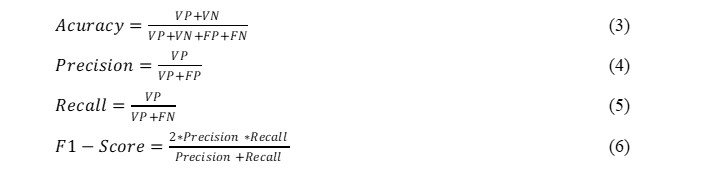Validation of results

To validate the results obtained in the simulations, a disc of the species Cenostigma pluviosum (Sibipiruna) was randomly chosen. In this disc, the conclusions of the research were applied in terms of measurement methodology, filter application, and number of measurement points. For the number of measurement points three conditions were applied – the number considered ideal (paper conclusion), a number above and a number below. The results of the confusion matrix metrics obtained in the real disc were compared to those obtained in the research (with the simulations).

RESULTS AND DISCUSSION

Wave Propagation Times Simulated for Image Formation

The number of theoretical routes that passed through the simulated defects varied as a function of the type of defect and as a function of the number of measurement points in the grid (6, 8, 10, 12, or 14).

Table 1 exemplifies the results obtained for the simulated propagation times obtained for simulated defect 1 (Fig. 2) in routes 1-4 and 2-8 of the mesh with eight measurement points in the contour (Fig. 3).

Table 1. Example of Results Obtained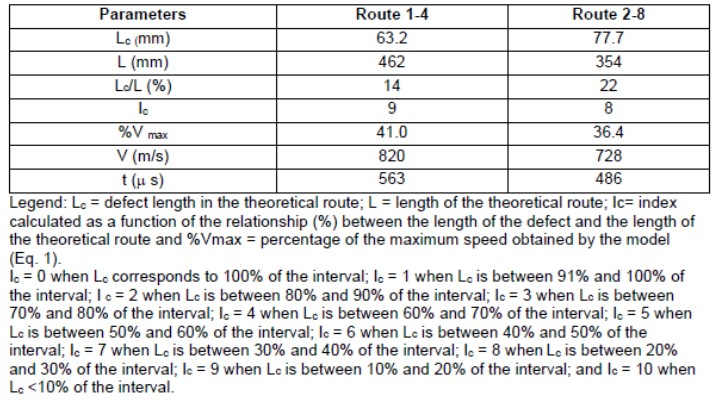Filters

After the iterative process of applying median filters, the best image results were obtained using 10 to 40 neighboring pixels. In practice, the average number of neighboring pixels obtained for each number of points of the measurement grid could be adopted. This average number (Table 2) was higher for the mesh with six measurement points (30 neighboring pixels) and remained fixed (20 neighboring pixels) from ten measurement points, a coherent result since greater interferences are expected in thicker meshes (with fewer measuring points). Figure 7 illustrates the improvement in image quality (reduction of interference) with the application of the filter.

Table 2. Number of Neighboring Pixels Used to Filter the Images Generated by the Inverse Square Distance (ISD)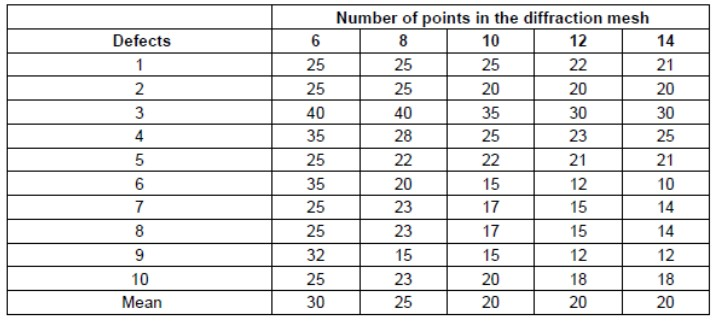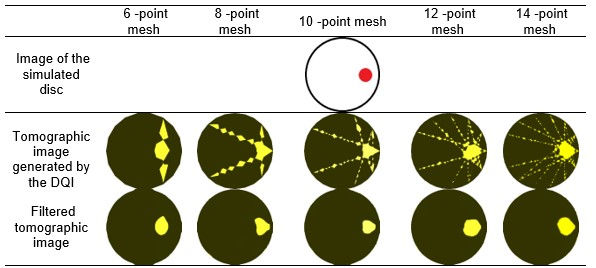Fig. 7. Example of images generated from the simulated disc (top) using the Inverse Square Distance (ISD) interpolation algorithm with diffraction grids of 6, 8, 10, 12 and 14 points in the contour (intermediate) and their respective images filtered using the median filter with 25 neighboring pixels (mesh with 6, 8 and 10 points), 22 neighboring pixels (mesh with 12 points) and 21 neighboring pixels (mesh with 14 points) (bottom).

Visual Analysis – Qualitative

Visually, the images produced using the DQI algorithm and cutting speed at 40% of the maximum speed for the identification of defective zones reasonably represented the simulated defects (Fig. 8), even for the mesh with the lowest number of measurement points. Very close defects (defect 5-Fig. 8), cannot be separated in the tomographic image, showing a general stain by the union of the defects. Considering the wave following the theoretical trajectory (straight), in the case of simulation 5 (Fig. 8), the edge tangential route passed through two defects, which caused a greater reduction in the wave propagation speed. According to the interpolator used, it is expected that the interference will form in the form of a bead (Palma 2017), which is not completely eliminated by the filter and causes the union between the defects to occur (Ostrovisky et al. 2017). It is also verified that the tomographic images do not represent the exact shape of the defect, especially in the case of defects with irregular shapes (defects 9 and 10-Fig. 8). For defect 10, which simulates a crack, reconstruction by interpolation is even more complex (Divos and Divos 2005; Feng et al. 2014; Strobel, et al. 2018).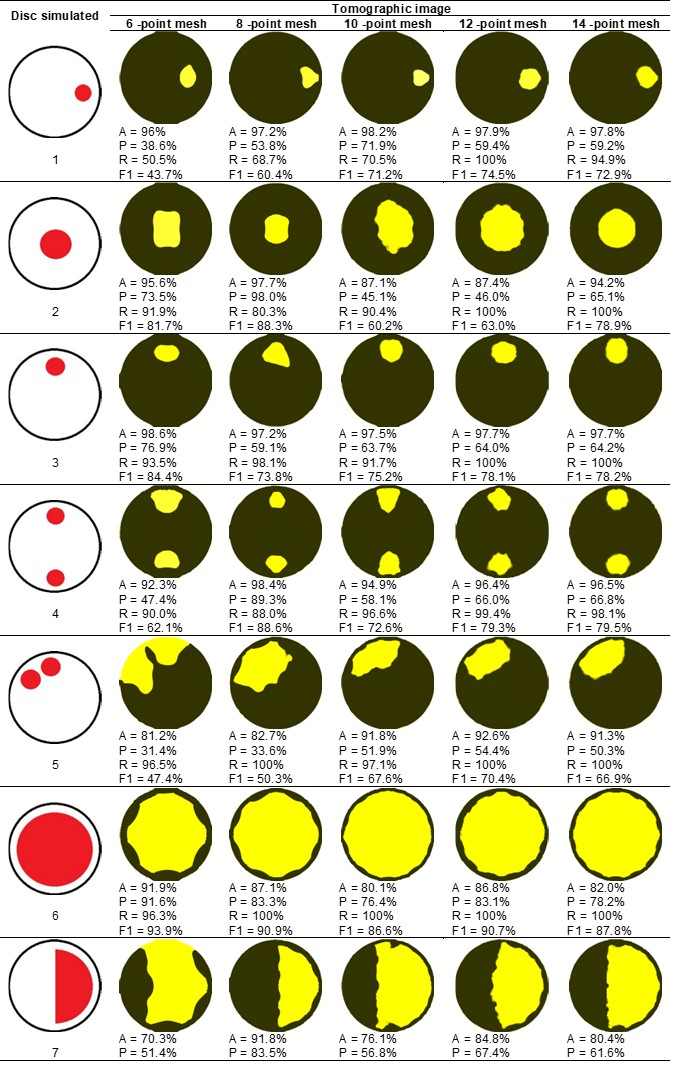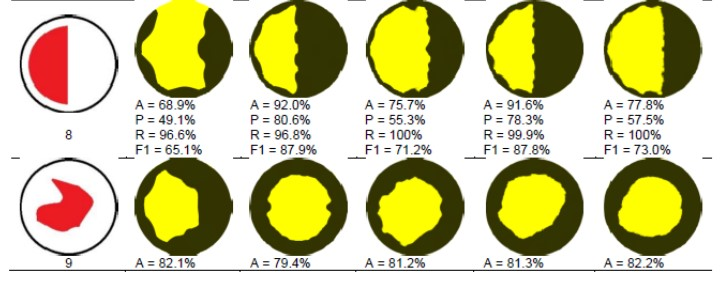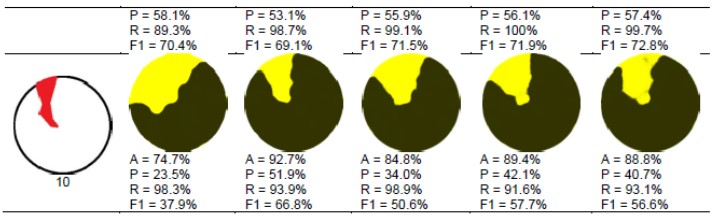Fig. 8. Discs with ten types of simulated defects, tomographic and metric images of the confusion matrix (accuracy (A), precision (P), recall (R) and F1-score (F1) resulting from diffraction grids with 6, 8, 10, 12 and 14 measurement points.

Analysis Using the Confusion Matrix – Quantitative

Quantitative analysis indicates that, considering the mean values obtained for the different simulated defects and different numbers of measurement points adopted, recall showed the best results for tomography (overall mean≅ 95%), followed by accuracy (overall mean≅ 89%), the F1-score (overall mean≅ 72%) and, finally, the accuracy (overall mean≅ 60%). Other researchers obtained similar results regarding the classification of metrics, with better results for recall and worse results for accuracy (Du et al. 2018).

Of the metrics of the confusion matrix, the accuracy represents the relationship between the observations that were correctly predicted (positive and negative) and the total number of observations (Eq. 3). In this paper, this metric indicates the relationship between the general correctness of the tomography in the detection of pixels in the zones with defects and in the zones without defects and all the points (pixels) that constitute the image (Eq. 3). Considering the means by defects, the worst results for accuracy were found for the largest defects (Fig. 8), and the best results were found for the smallest defects (Fig. 8). The reference literature of this methodology (Luque et al. 2019) indicates that the accuracy is affected when there is an imbalance between the positive and negative zones. In the case of this study, this occurs for small defects, since the positive zone (PV) is very small compared to the zone without defect (NV). Disregarding the type of defect, in the real case of an inspection where the internal condition of the stem is unknown, the best accuracy results (greater than 90%) were obtained for eight points (91.6%) and 12 points (90.6%) of measurement in the contour. Although the highest accuracy was obtained for eight and 12 points, for six measurement points, the mean result (85.1%) was only 7% lower than the best accuracy, confirming the visual observation that all the images produced made it possible to infer, in a general and approximate manner, the simulated defects.

In this study, precision is the relationship between correctly predicted positive observations (i.e., correctly predicted defects) and the total number of positive observations (true or false) (Eq. 4). Thus, this metric has higher values when the image does not infer positive points (defects) out of place (FP), even if the dimension of the defective zone is smaller in the image than in the real condition. For this metric, the use of eight measurement points generated the best result (Fig. 8), followed by 12 points (10% worse) and 14 points (12% worse). This metric, in addition to having the worst overall mean, was also the one with the highest coefficients of variation among the different types of defects (from 16.7% for 14 points to 39.3% for 6 points).

Recall is obtained by the relationship between correctly predicted positive observations (in the case of this study, the correctly predicted defective zones) and all real positive observations, including the pixels that are positive in the real image and were inferred as negative, i.e. the false negatives (Eq. 5). Thus, in this case, the metric verifies that the dimension of the defect is not smaller than the real dimension; that is, it considers the false negatives that are the pixels where the image should have shown a defect but did not. This metric was the only one with 100% values for some types of defects from eight measurement points (Fig. 8). For this metric, the best results were obtained for 12 measurement points (99.1%), with 100% accuracy for 70% of the types of defects (Fig. 8). Any number of points (six to 14) had values greater than 90% for recall, and the variability in the relation to the type of defect, measured by the coefficient of variation (between 2.6% for 14 points and 15.8% for six points), was the lowest among all metrics, with a mean of 8.4%. It is important to note that the problem with this metric is that because it does not consider false positives, the values can be high or even 100% even when the image is filled with the zone of interest, showing a defect of dimensions much larger than the real one.

The F1-score (Eq. 6) is a metric that indicates a balance between precision and recall; therefore, the result (Table 3) was a mixture between the correctness of the size and position of the defects. Thus, for this metric, the best number of measurement points was eight (76.4%), followed by 12 with a very close value (75.4%) and 14 (74.3%) (Fig. 8). The coefficient of the mean variation for the case of this metric, considering all types of defects, was 17%, ranging from 11.2% (14 points) to 28% (6 points).

Because there is no prior knowledge of the type of defect, a condition for the inspection of a tree is considering all types of mixed defects. As such, the statistical analysis of comparison of means (with 95% confidence level) shows that there was a significant difference between the results obtained with different numbers of measurement points (Fig. 11) only for the recall metric. In this case, the recall for 12 and 14 measurement points differed statistically from that obtained for six measurement points. For the other metrics, the results were statistically equivalent because the variability around the mean prevented the detection of significant differences (Fig. 9, 10 and 12).

Numerically, the adoption of eight measurement points allowed for the conditions of the simulated discs to obtain the best mean values of accuracy, precision, and F1-score, while 12 points had the best recall value. When adopting eight measurement points, the loss of recall was 7%, while when adopting 12 measurement points, the loss of precision was 10%, and of accuracy and F1-Score was 1% each (Table 3).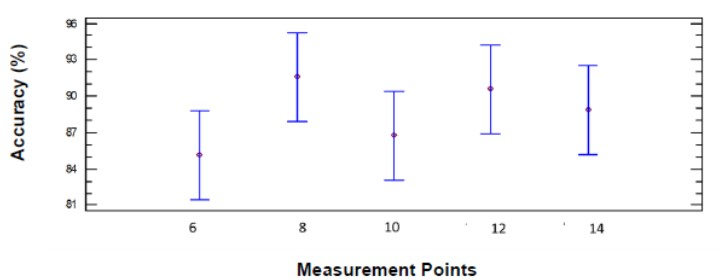Fig. 9. Statistical analysis of comparison of means of accuracy obtained in ultrasonic tomography for different measurement points in the diffraction mesh